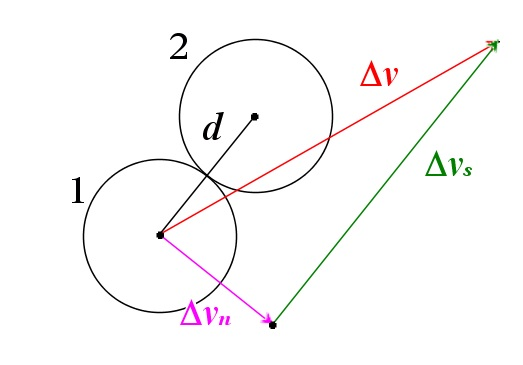# Colliding non-rotating balls in 2D

Up a level : Themodynamics
Previous page : A simulation of an Atmosphere
Next page : Grouping the particlesIn 1D a totally elastic collision between two balls is rather easy to figure out, but how about in 2D?

You will find a demo of this here.

We assume that we have no friction between the balls, so all the forces acting must be normal forces, and thus the collisions cannot cause any rotation of the balls. Let us start by assuming that one of the balls is initially at rest.  We can shift our perspective, and our frame of reference, by subtracting the x-and y– components of the velocity of the second particle from both. In that way the second will be at rest in the new frame of reference. We have$\Delta {v_x} = {v_{1x}} - {v_{2x}},\quad \Delta {v_y} = {v_{1y}} - {v_{2y}}$

Or, written as vectors,$\Delta {{\bf{v}}_f} = {{\bf{v}}_1} - \Delta {{\bf{v}}_s}$

The forces acting on the two particles as they collide will be along the axis connecting their midpoints for the reasons mentioned above.The acceleration, and thus the changes in velocity must hence be parallel to the line connecting the two midpoints.

We may now split Δv in two components, one parallel to the forces, and one perpendicular, as in the figure. The Δvs will now be the change in the velocity of the second particle, and since we must conserve momentum, we must change the velocity of the second particle by the same amount, but in the opposite direction. It will thus, in our frame of reference, end up with the velocity$\Delta {{\bf{v}}_f} = {{\bf{v}}_1} - \Delta {{\bf{v}}_s}$

The second particle was initially at rest so that it would end up with the velocity Δvs.We can here see that the kinetic energy is conserved too since we have that the end velocities are perpendicular to each other, so by Pythagoras’s, we get$\begin{array}{l} {E_k} = \frac{1}{2}m{\left( {\Delta v} \right)^2} = \frac{1}{2}m{\left( {\sqrt {{{\left( {\Delta {v_f}} \right)}^2} + {{\left( {\Delta {v_s}} \right)}^2}} } \right)^2}\\ \quad = \frac{1}{2}m\left( {{{\left( {\Delta {v_f}} \right)}^2} + {{\left( {\Delta {v_s}} \right)}^2}} \right) \end{array}$

Ok, so we need to find Δvs. This is basically the projection of Δvonto the line connecting the two centres of the particles. We can here use a lot of algebra, or, if we know the scalar product we have that${\bf{d}} \cdot \Delta {\bf{v}} = d\Delta v\cos \theta = {d_x}\Delta {v_x} + {d_y}\Delta {v_y}$

where θ is the angle between d and Δv. What we are looking for is$\Delta {v_s} = \Delta v\cos \theta = \frac{{{d_x}\Delta {v_x} + {d_y}\Delta {v_y}}}{d} = \frac{s}{d}$

where$s = {d_x}\Delta {v_x} + {d_y}\Delta {v_y}$

This will give us the magnitude. What we also know is that Δvf is parallel to d. This will enable us to find the x– and y-components of Δvf by using the similar triangle made by d, Δdx, and Δdy.

By similarity we get$\frac{{\Delta {v_{sx}}}}{{\Delta {v_s}}} = \frac{{\Delta x}}{d},\quad \frac{{\Delta {v_{sy}}}}{{\Delta {v_s}}} = \frac{{\Delta y}}{d}$

or$\Delta {v_{sx}} = \frac{{\Delta x}}{d}\Delta {v_s},\quad \Delta {v_{sy}} = \frac{{\Delta y}}{d}\Delta {v_s}$

Combining this with the equation$\Delta {v_s} = \frac{s}{d}$

we get,$\Delta {v_{sx}} = \frac{{\Delta x}}{{{d^2}}}s,\quad \Delta {v_{sy}} = \frac{{\Delta y}}{{{d^2}}}s$

These values are thus the changes in velocities for the two particles. We add these to the components of the initial velocity of particle 2 and subtract it from the initial velocity of particle 1 – and we now have the velocities of the particles after the collision.

Let us look at a bit of JavaScript that uses this.

Here we have used the result of the dot products in two ways. The first is to use it to determine if the particles are moving toward each other, so that will have a collision, or away from each other. If the vector Δvs is pointing in the opposite direction from the vector from particle 1 to particle 2 then the dot-product is negative, and the particles are moving away from each other. If the dot-product is positive, we proceed with the calculations described above.Up a level : Themodynamics
Previous page : A simulation of an Atmosphere
Next page : Grouping the particlesLast modified: Nov 26, 2023 @ 19:46